# Calculation of the cubic capacity of sawn timber - edged and unedged boards

In the process of construction of wooden structures used various types of lumber. Before its acquisition there is a need for carrying out calculations. At the same time, the main problem is that, due to inconsistent characteristics and quality of the goods, it is sometimes very difficult to correctly calculate the volume of sawn timber.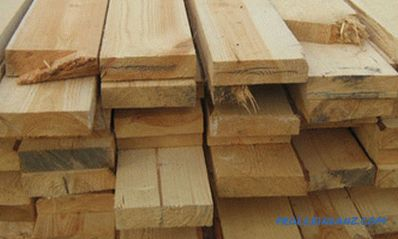In order not to buy excess lumber when building a house, you need to know how to calculate its cubic capacity.

In some cases, lumber from the manufacturer is supplied in packages that accurately indicate the volume and value of the goods. But this practice is very rare. Therefore, the question of how to calculate the cubic capacity of a particular type of wood today is quite relevant.

## Features of calculating the cubic capacity of lumber

Today, you can buy wood at any logging company, and a huge selection of finished products allows you to choose the best option for carrying out certain tasks. If the width of the longitudinal sawing wood is more than 100 mm, then it is called a timber. This building material has the following standard dimensions: 100x100, 100x150, 150x150, 180x180 mm with a length of 600 cm. If the thickness of the kerf differs from the width by more than 2 times, then such a building material is called a board, which can be either edged or uncut.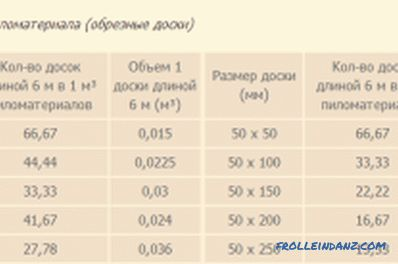Table for calculating edged boards.

The degree of wood processing, its type and type affect the calculation of cubic capacity. Quite often a situation arises when there is a different amount of both edged and unedged boards in 1 m 3 building material. For calculations, you need to choose the method by which this operation will be carried out. The first method consists in measuring the dimensions of each element with their subsequent multiplication and summation. The second is the use of special tables (cubic tables).

## Calculation of cubic capacity of trimmed lumber

To deal with all the subtleties of the calculation, an example will be given in which you need to calculate the volume of timber required for the construction of a wooden house 6x6 m with a ceiling height of 230 cm. use a bar with a section of 15x15 cm and a length of 600 cm.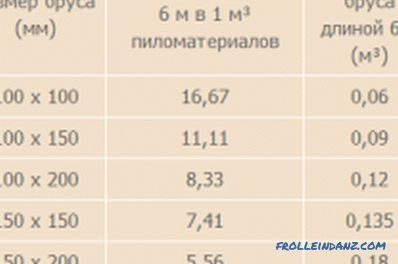Calculation table for a clean-cut timber.

First you need to know the necessary number of logs (excluding windows and doors) that will go to the construction of walls. For this, the height of the building should be divided by the height of one log: 230/15 = 15.33 or 15 pcs. That is, for the construction of one wall will need 16 bars (15 + 1 base crown). After that, the resulting value is multiplied by 4 walls: 16 * 4 = 64 pcs. Next you need to know how much it will be in m 3 : 64 * 0.15 * 0.15 * 6 = 8.64 m 3 .

To facilitate calculations, you can use the data from a special table. Using these values, you can check the result obtained earlier. According to the table, the volume of 1 beam 15x15 cm will be equal to 0.135 m 3 . Therefore, the cubic capacity of 64 such elements will be equal to: 64 * 0.135 = 8.64 m 3 .

## Calculation of cubic capacity of unedged lumber

Unedged board is one of the simplest types of sawn timber, which is widely used in cases where the appearance of an object does not matter. This building material is a longitudinally sawn log with wane. Unlike edged sawn timber, unedged board has only a fixed thickness and length, and the width can vary depending on the location from which the log is cut to a specific element. Therefore, the calculation of cubic capacity for unedged boards is carried out by another method.

For the most accurate calculation, the boards are folded in one stack, after which the width of the widest and narrowest element is measured. Further, the obtained values ​​are summed and divided by 2. As a result, the average width of one element will be obtained. After that, the width, thickness, and length of the stack are multiplied together, and then the reduction factor is applied.

According to GOST, for unedged lumber, the reduction factor will be 0.63, and for a half-timber, 0.5.

For example, you need to calculate the volume of 150 unedged boards if the thickness of one element is 25 mm and the length is 600 cm. First, 10 boards are stacked, after which the width is measured widest and narrowest element. For example, the widest board has a width of 21 cm, and the narrowest one is 16 cm. Then these values ​​are summed up and divided by 2: (21 + 16) / 2 = 18.5 cm. This will be the average width.

Next, the volume of the received stack is calculated: 0.025 * 10 * 0.185 * 6 * 0.63 (reduction factor) = 0.175 m 3 . After that, another stack is added and its volume is calculated. At the last stage, the volumes of each stack are summed, which will be the total cubic capacity of the unedged board.

•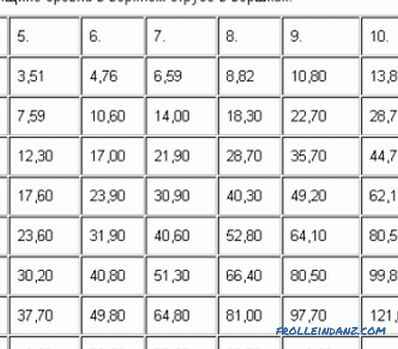Phased calculation of lumber

Calculation of lumber and its subtleties. What are the most commonly used lumber and how to distinguish them? The phased description of the compilation of the calculation table. Additional nuances of calculation.

•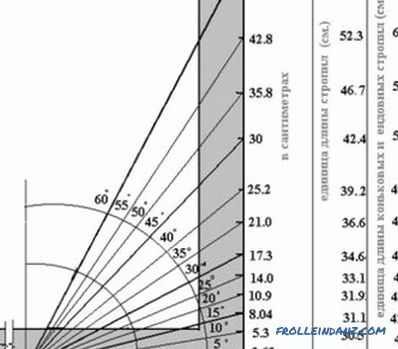How to calculate the length of rafters correctly?

Recommendations on how to calculate the length of the rafters, taking into account the shape of the roof, wind and snow loads. An example of calculating the cross section of a timber and its length. Possible errors in the work.